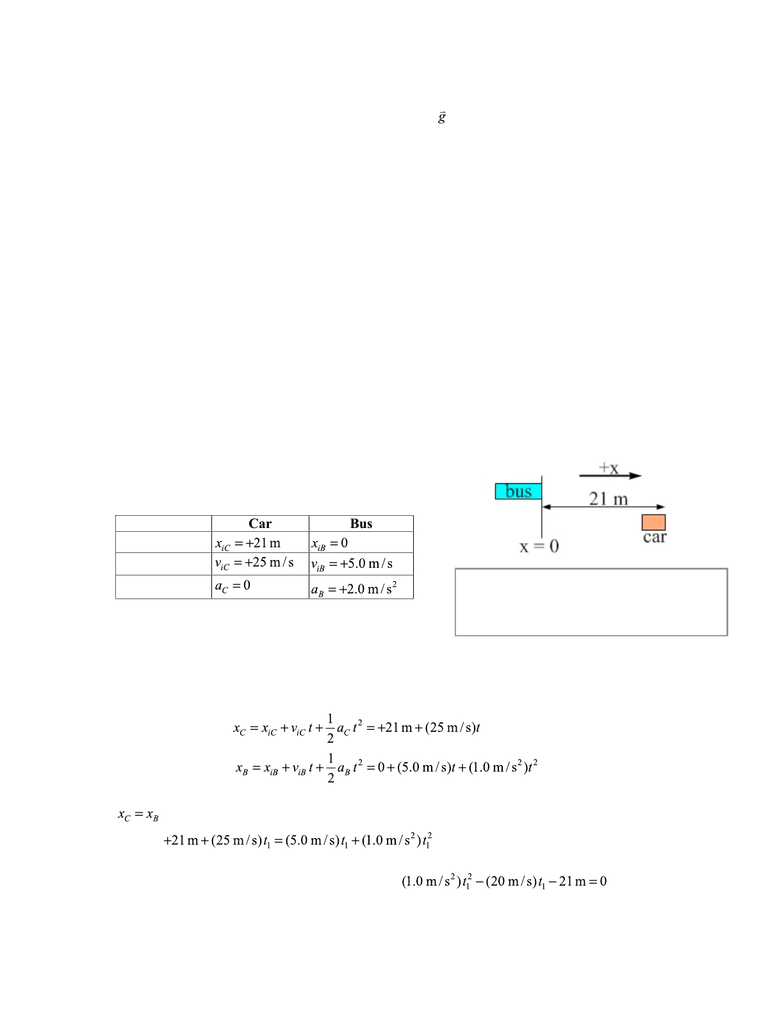# CAS PY 211 Lecture Notes - Lecture 2: Minor Places In Arda, One Direction, Quadratic Equation

212 views3 pages
School
Boston University
Department
Physics
Course
CAS PY 211
ProfessorAnswer to Essential Question 2.7: A common misconception is that the ball’s acceleration is zero
at the maximum-height point. In fact, the acceleration is , 9.8 m/s2 down, during the entire trip.
This is what is shown on the acceleration graph, for one thing – the graph confirms that nothing
special happens to the acceleration at t = 2.0 s, even though the ball is momentarily at rest. One
reason for this is that the ball is under the influence of gravity the entire time.
2-8 Solving Constant-Acceleration Problems
Consider one more example of applying the general method for solving a constant-
acceleration problem.
EXAMPLE 2.8 – Combining constant-acceleration motion and constant-velocity motion
A car and a bus are traveling along the same straight road in neighboring lanes. The car
has a constant velocity of +25.0 m/s, and at t = 0 it is located 21 meters ahead of the bus. At time t
= 0 , the bus has a velocity of +5.0 m/s and an acceleration of +2.0 m/s2.
When does the bus pass the car?
SOLUTION
1. Picture the scene – draw a diagram. The diagram in Figure 2.21 shows the initial
situation, the positive direction, and the origin. Let’s choose the positive direction to
be the direction of travel, and the origin to be the initial position of the bus.
2. Organize the data. Data for the car and the bus is
organized separately in Table 2.3, using subscripts
C and B to represent the car and bus, respectively.
Table 2.3: Summarizing the information that was given
about the car and the bus.
3. Solve the problem. Let’s use Equation 2.8 to write expressions for the position of
each vehicle as a function of time. Because we summarized all the data in Table 2.3
we can easily find the values of the variables in the equations.
For the car: .
For the bus: .
The bus passes the car when the vehicles have the same position. At what time does
? Set the two equations equal to one another and solve for this time (let’s call it t1).
Bringing everything to the left side gives: .
Chapter 2 – Motion in One Dimension Page 2 - 16
Figure 2.21: A diagram showing the initial
positions of the car and the bus, the position
of the origin, and the positive direction.
Car
Bus
Initial position
Initial velocity
Acceleration
Unlock document

This preview shows page 1 of the document.
Unlock all 3 pages and 3 million more documents.